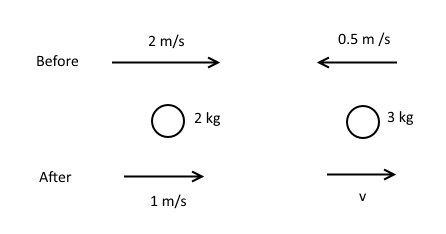Momentum and impulse

Introduction

You might have been in the following scenario before. Image you're a passenger in a car going at a good speed, say 60mph. All of a sudden a hazard appears on the road and the driver is forced to brake abruptly.

You feel your whole body pressing forwards against your seatbelt, and you feel the pressure in your head and hands. It's very uncomfortable for the few seconds the brakes are applied.

That's because your body had momentum in the same direction of travel as the car did until the driver applied the brakes.

Momentum is a vector quantity defined by the following formula

$$\mathbf{p} = m\mathbf{v}$$

Where $\mathbf{p}$ is linear momentum measured in $\textrm{kgms}^{-1}$ or Ns (Newton seconds), $m$ is the mass of the object whose momentum we're measuring in kg, and $\mathbf{v}$ is the velocity of the object in $\textrm{ms}^{-1}$. Momentum and velocity are always in the same direction.

Conservation of linear momentum

In M1 the questions about momentum always involve the following principle:

In a closed system if no external forces are applied then the total amount of linear momentum in it doesn't change.

Put into maths terms, image a collision between two objects with masses $m_1$ and $m_2$ respectively. If their velocities before the collision were $u_1$ and $u_2$, and after the collision became $v_1$ and $v_2$, then

$$m_1 u_1 + m_2 u_2 = m_1 v_1 + m_1 v_2$$

Two particles labelled 1 and 2 interact. By Newton's third law, the forces between them are equal and opposite. By Newton's second law

$$F_1 = \frac{dp_1}{dt} \textrm{ and } F_2 = \frac{dp_2}{dt}$$

Therefore

$$\frac{dp_1}{dt} = -\frac{dp_2}{dt}$$

$$\Rightarrow \frac{d}{dt}\left(p_1 + p_2\right) = 0$$

The total amount of linear momentum in a system does not vary with time.

In an wordy way that might be more intuitive

Total momentum before = Total momentum after

Example

Q) A particle with mass 2kg and another particle with mass 3kg travel in the same but in opposite directions so that they eventually collide. If before the collision their respective velocities were $2\textrm{ms}^{-1}$ and $0.5\textrm{ms}^{-1}$, and that after the collision the first particle keeps on moving in the same direction with halved velocity, and the second particle moves in the opposite direction, find the velocity of the second particle after the collision.

A) Firstly draw the diagram with the correct directions marked on.By CLM we know that the total momentum is the same before and after the collision. That doesn't mean simply adding them up though. You have to pick a direction and stick with it for the calculation, so velocities in opposite directions are negative. I'll use right for positive here.

$$\left(2 \times 2\right) + \left(3 \times \left(-0.5\right)\right) = \left(2 \times 1\right) + \left(3 \times v\right)$$

$$4-1.5 = 2 + 3v$$

$$\therefore v = \frac{1}{6} = 0.167 \textrm{ms}^{-1} \left(\textrm{3.s.f.}\right)$$

Impulse

The impulse of an object over a time period is its change in momentum over that time period.

Impulse = (final momentum) - (initial momentum)

In maths terms we say

$$\mathbf{I} = \Delta \mathbf{p} = \mathbf{p}_{\textrm{after}}-\mathbf{p}_{\textrm{before}}$$

Where $\mathbf{I}$ is the impulse, measured in Ns or $\textrm{kgms}^{-1}$, and $\Delta \mathbf{p}$ is the change in momentum, measured in the same units. The impulse and change in momentum are always in the same direction.

Strictly speaking, impulse is defined as the integral of a resultant force $\sum\mathbf{F}$ over the time $t$ that it's applied. In M1 things are a bit simpler. We note from Newton's second law that

$$\sum \mathbf{F} = \frac{d\mathbf{p}}{dt}$$

$$\Rightarrow \left(\sum \mathbf{F}\right)dt = d\mathbf{p}$$

Simplifying down we say that

$$\mathbf{F}t = \Delta \mathbf{p} = \mathbf{I}$$

So a resultant force applied for a long time has a large impulse. It is also where the units 'Ns' come from.

Conservation

Like momentum, impulse is conserved in a closed system. That means that when two objects collide, they exert the exact same magnitude of impulse on each other for the duration of the collision. Bear in mind it's the magnitudes that are always the same - they are in opposite directions.

That means that you can also compare impulses in questions like my example above. It's the same principle and perfectly valid.

In an M1 exam on collisions like the one above, you will almost always be asked to find the impulse that one object exerts on the other object. Calculate the change in momentum on the subject object.

Remember that like momentum, you have to pick a direction and stick with it for the calculation, because impulse is a vector too.

Example

Q) In the same situation as the above example, find the magnitude of the impulse applied by the first particle on the second particle.

A) Impulse and change in momentum are always in the same direction, so we'll pick right as positive.

$$\textrm{Impulse} = \left(\textrm{final momentum}\right) - \left(\textrm{initial momentum}\right)$$

$$\mathbf{I} = \left(3\times \frac{1}{6}\right) - \left(3\times \left(-0.5\right)\right)$$

$$\mathbf{I} = 3\left(\frac{1}{6} + 0.5\right) = 2 \textrm{Ns}$$

And indeed it is positive so it acts from left to right as per our resolving direction, which makes sense when you visualise object one exerting an impulse onto object two.

Showing that we can analyse the first object and get the same magnitude due to conservation of impulse, picking right as positive again

$$\mathbf{I} = \left(2\times 1\right) - \left(2\times 2\right)$$

$$\mathbf{I} = 2\left(1-2\right) = -2 \textrm{Ns}$$

And obviously $|-2| = 2\textrm{Ns}$.

What you have just calculated is the impulse exerted by object two on object one. It's negative and we were resolving in the right hand direction, so it must go from right to left.